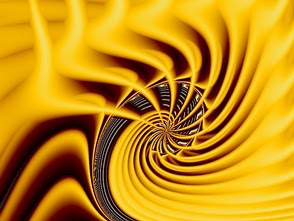## September 25, 2004

### Superconductors and induced current

A very short summary of super-conductors: they are conductors that provides no resistance to a current flowing through them. Despite the rather simplified explanation, they are an extremely complex study in quantum mechanics and a whole slew of scientific research besides. But for our purposes, the above definition shall suffice.

Now I'll introduce Ohm's law:
V=IR .... (1)
V is voltage, I is current, R is resistance. So, given zero resistance in a super-conductor, one would logically expect therefore, to never measure a voltage across a superconductor, even if you injected an infinite amount of current. On a side note, superconductor have what is called a critical current above which they cease to be superconductors.

And the last equation for the night, is one that gives the electric field (E) induced by a changing magnetic field, (B).
E = -dB/dt ... (2)
Simply put, E is the negative gradient of B over time. Now here is what I am puzzling over:
1. Let there be a changing B around a superconductor loop
2. By (2) this would produce an electric field, and thus a voltage, in the superconductor loop
3. Yet by (1) this is impossible, because anything multiplied by 0 gives 0
What it means if the following:
1. I have made a mistake somewhere
2. Ohm's law doesn't hold for superconductors.
Its most likely 1 is true :) Either way, here are more questions

1. So what does happen when you try place a superconductor in a changing magnetic field?
2. What would you measure?
Oh, and here is a fractual piCheers,
Steve

1.2.